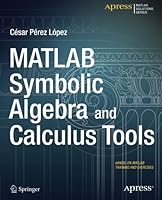# MATLAB Symbolic Algebra and Calculus Tools0 Reviews
2014-12-09
260 pages

## Book Description

is a high-level language and environment for numerical computation, visualization, and programming. Using , you can analyze data, develop , and create models and applications. The language, tools, and built-in math enable you to explore multiple approaches and reach a solution faster than with spreadsheets or traditional programming languages, such as C/C++ or Java.

MATLAB Symbolic and Calculus Tools introduces you to the MATLAB language with practical hands-on instructions and results, allowing you to quickly achieve your goals. Starting with a look at symbolic variables and functions, you will learn how to solve equations in MATLAB, both symbolically and numerically, and how to simplify the results. Extensive coverage of polynomial solutions, inequalities and systems of equations are covered in detail. You will see how MATLAB incorporates vector, matrix and character variables, and functions thereof. MATLAB is a powerful symbolic manipulator which enables you to factorize, expand and simplify complex algebraic expressions over all common fields (including over finite fields and algebraic field extensions of the numbers). With MATLAB you can also work with ease in matrix algebra, making use of commands which allow you to find eigenvalues, eigenvectors, determinants, norms and various matrix decompositions, among many other features. Lastly, you will see how you can use MATLAB to explore mathematical analysis, finding limits of sequences and functions, sums of series, integrals, derivatives and solving differential equation.

### What youll learn

• How to use MATLAB to work with numeric and symbolic variables, including vector, matrix and character variables
• How to use MATLAB to handle polynomials and general algebraic expressions, factorizing, expanding and simplifying over a wide range of fields
• How to use MATLAB to work on matrix and vector functions, including all the standard matrix operations and decompositions
• How to solve equations and systems of equations using MATLAB
• How MATLAB can be used to explore mathematical analysis, by finding limits of sequences and functions, sums of series, integrals, derivatives, and solving differential equations

### Who this book is for

This book is for anyone who wants to work in a practical, hands-on manner on symbolic algebra or calculus problems with MATLAB. You'll already have a core understanding of undergraduate level calculus, algebra and , and have access to an installed version of MATLAB, but no previous experience of MATLAB is assumed.

Chapter 1: Symbolic Variables and Functions
Chapter 2: Algebraic Expressions
Chapter 3: Polynomial Divisibility
Chapter 4: Symbolic Matrix Algebra
Chapter 5: Equations and Systems
Chapter 6: Series, Continuity, Integrals and Differential Equations

## Book Details

• Title: MATLAB Symbolic Algebra and Calculus Tools
• Author:
• Length: 260 pages
• Edition: 1
• Language: English
• Publisher:
• Publication Date: 2014-12-09
• ISBN-10: 1484203445
• ISBN-13: 9781484203446